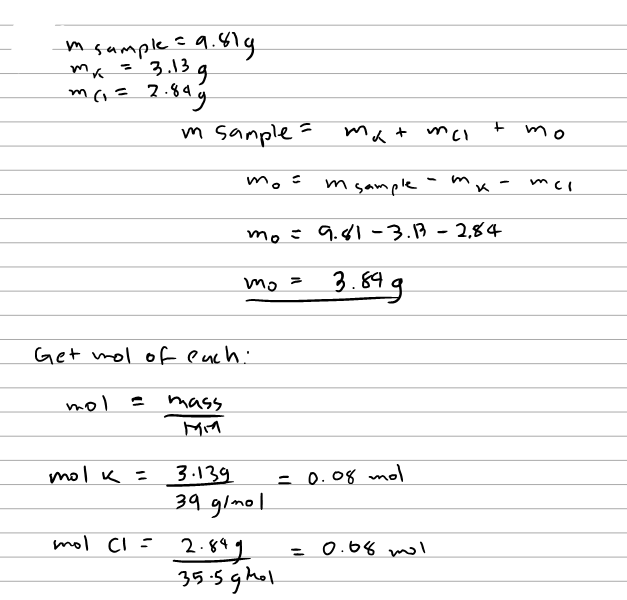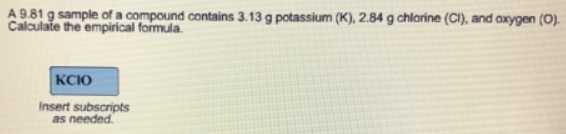# Problem: A 9.81 g sample of a compound contains 3.13 g potassium (K), 2.84 g chlorine (Cl), and oxygen (O). Calculate the empirical formula.

###### FREE Expert Solution87% (364 ratings)###### Problem Details

A 9.81 g sample of a compound contains 3.13 g potassium (K), 2.84 g chlorine (Cl), and oxygen (O). Calculate the empirical formula.